Question of The Day09-06-2022

Two men standing 15 km apart on a highway started to walk toward each other. First man walks for 2.5 km straight then take a right turn and walks for 1.5 km. He then turns left and walks for another 2.5 km and then takes the direction back to reach the highway. In the meantime, the other man can only travel 3.5 km along the highway. What would be the distance between them at this point? (in km.)

Correct Answer : a ) 6.5

Explanation :

Let’s visualize the information given in the question by drawing the diagram step by step.

Step 1: Two men standing 15 km apart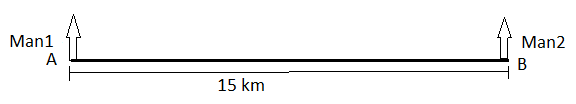Step 2: Let's draw the figure of Man1’s walk step by step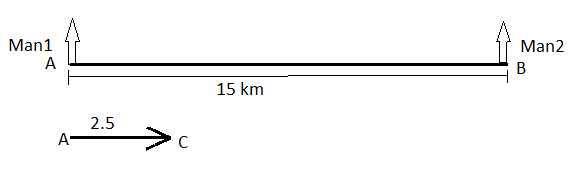Step 3: After reaching C Man1 took a right turn and walked for 1.5 km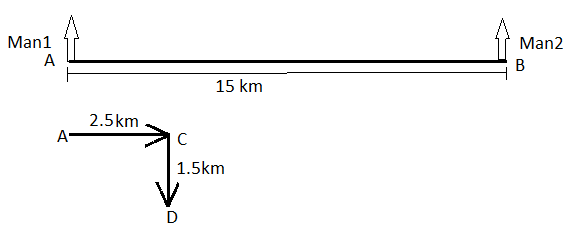Step 4: Now, after reaching D Man1 took a left turn and walked for 2.5 km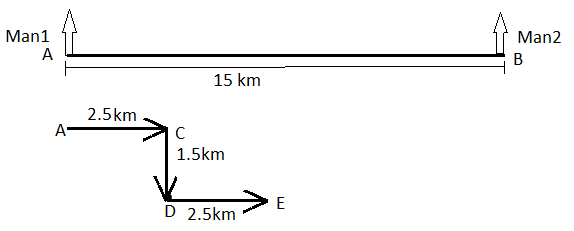Step 5: After reaching E, Man1 took the direction towards the highway and walked 1.5 km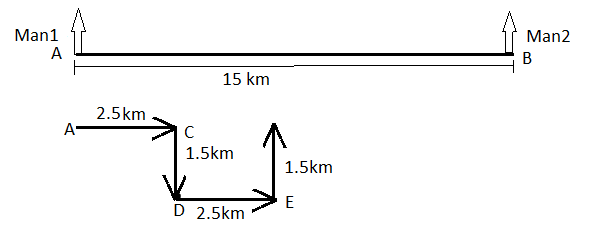Step 6: Now, draw the walk of Man2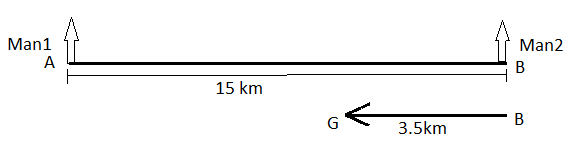Combining the diagram of Man1 and Man2’s walk together.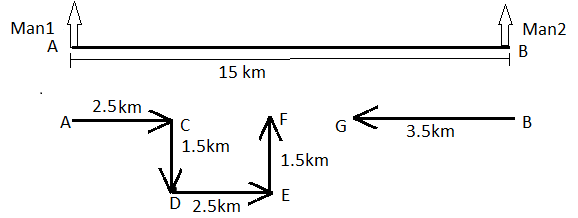Required distance FG=AB-AC+CF+GB

⇒FG=15-2.5+2.5+3.5

⇒FG=6.5 km

Therefore, the distance between Man1 and Man2 is 6.5 km.

Hence, A is the correct answer.1